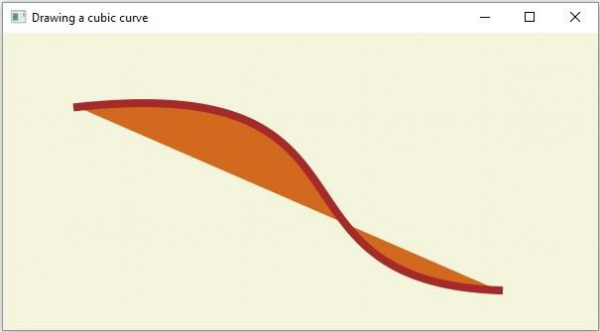# How to create a CubicCurve using JavaFX?

A cubic curve is a third-degree polynomial function of two variables.

In JavaFX a cubic curve is represented by the javafx.scene.shape.CubicCurve class. This class contains eight properties they are −

• startX − This property represents the x coordinate of the starting point of the curve. You can set the value to this property using the setStartX() method.

• startY − This property represents the y coordinate of the starting point of the curve. You can set the value to this property using the setStartY() method.

• controlX1: This property represents the x coordinate of the first control point of the curve. You can set the value to this property using the setControlX1() method.

• controlY1 − This property represents the y coordinate of the first control point of the curve. You can set the value to this property using the setControlY1() method.

• controlX2 − This property represents the x coordinate of the second control point of the curve. You can set the value to this property using the setControlX2() method.

• controlY2 − This property represents the y coordinate of the second control point of the curve. You can set the value to this property using the setControlY2() method.

• endX − This property represents the x coordinate of the endpoint of the curve. You can set the value to this property using the setEndX() method.

• endY − This property represents the y coordinate of the endpoint of the curve. You can set the value to this property using the setEndY() method.

To create a circle you need to −

• Instantiate this class.

• Set the required properties using the setter methods or, bypassing them as arguments to the constructor.

• Add the created node (shape) to the Group object.

## Example

import javafx.application.Application;
import javafx.scene.Group;
import javafx.scene.Scene;
import javafx.scene.paint.Color;
import javafx.stage.Stage;
import javafx.scene.shape.CubicCurve;
public class DrawingCubicCurve extends Application {
@Override
public void start(Stage stage) {
//Drawing a cubic curve
CubicCurve cubicCurve = new CubicCurve();
//Setting properties to cubic curve
cubicCurve.setStartX(75.0f);
cubicCurve.setStartY(75.0f);
cubicCurve.setControlX2(250.0f);
cubicCurve.setControlY2(250.0f);
cubicCurve.setControlX1(400.0f);
cubicCurve.setControlY1(40.0f);
cubicCurve.setEndX(500.0f);
cubicCurve.setEndY(260.0f);
//Setting other properties
cubicCurve.setFill(Color.CHOCOLATE);
cubicCurve.setStrokeWidth(8.0);
cubicCurve.setStroke(Color.BROWN);
//Setting the scene object
Group root = new Group(cubicCurve);
Scene scene = new Scene(root, 600, 300);
stage.setTitle("Drawing a cubic curve");
stage.setScene(scene);
stage.show();
}
public static void main(String args[]){
launch(args);
}
}

## Output